# Neural Network Simplified

In this post we will understand basics of neural network.

Prerequisite for the blog is basic understanding of machine learning and it would be good if you have tried a few machine learning algorithms

First a brief introduction to Artificial Neural Networks also called as ANN.

Inspiration for a lot of Machine Learning algorithm comes from nature and biggest inspiration is our brain, how we think, learn and take decisions.

It is interesting to understand when we touch something hot how the neurons in our body transmit signal to the brain. Brain then produces impulses to withdraw from the hot area. We have got trained based on the experience. Based on our experience we started taking better decisions.

Using the same analogy, when we send an input to a neural network (touching a hot substance) then based on the learning(previous experiences) we produce an output(withdraw from the hot area). In future when we get a similar signal(touching a hot surface) we can predict the output(withdraw from hot area).

Let’s say we have inputs like temperature, wind speed, visibility, humidity to predict what kind of weather we will have — Rainy, Cloudy, or Sunny.

This can be represented as shown below.

let’s represent this using a neural network and understand the components of a neural network.

A neural network receives input, converts the input signal by changing the state using an activation function to produce an output.

Output will change based on the input received, strength if the signal represented by weights and the activation applied to the input parameters and weights.

A neural network is very similar to the neuron in our nervous system.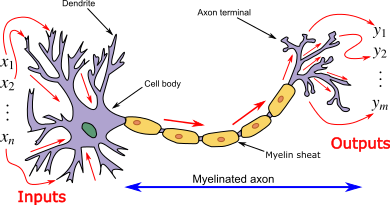Image source : wikipedia

x1, x2, …xn are input signal to dendrites, there will be a change of state to produce output y1, y2,…yn at the axon terminal of the neuron.

Taking our example of predicting weather, where temperature, wind speed, visibility and humidity are input parameters. These inputs are then processed by a neuron by applying weights on the input using an activation function to produce an output . Here the predicted output is the type of weather- sunny, rainy, or cloudy.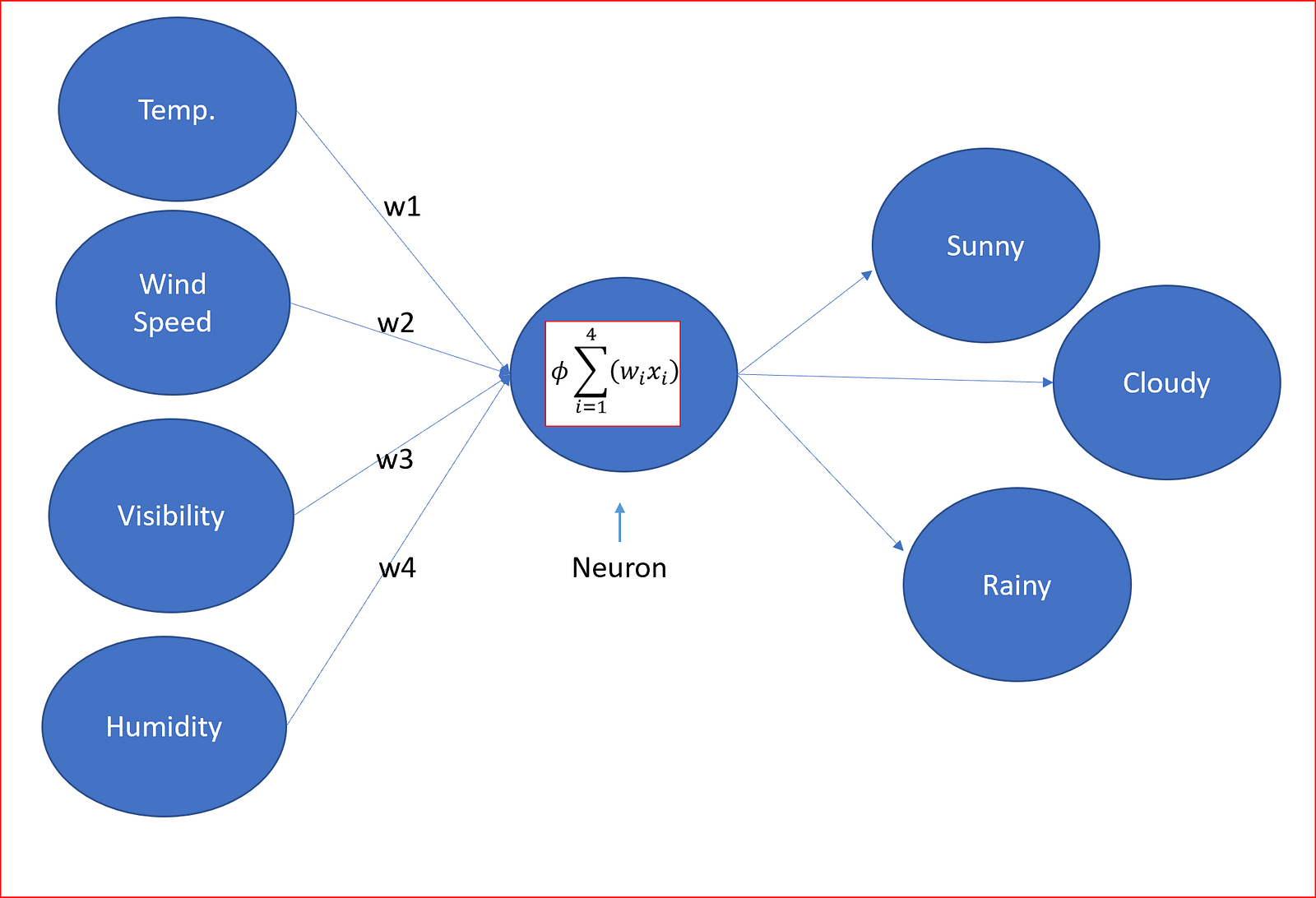w1, w2, w3 and w4 are the weights applied to the neurons and ɸ is the activation function.

so, what are the components of a neural network

A neural network will have

• Input layer, with the bias unit which is 1. It is also referred as the intercept.
• One or more hidden layers, each hidden layer will have a bias unit
• Output layer
• Weights associated with each connection
• Activation function which converts an input signal of a node to an output signal

Input layer, hidden layer and output layers are usually referred as dense layers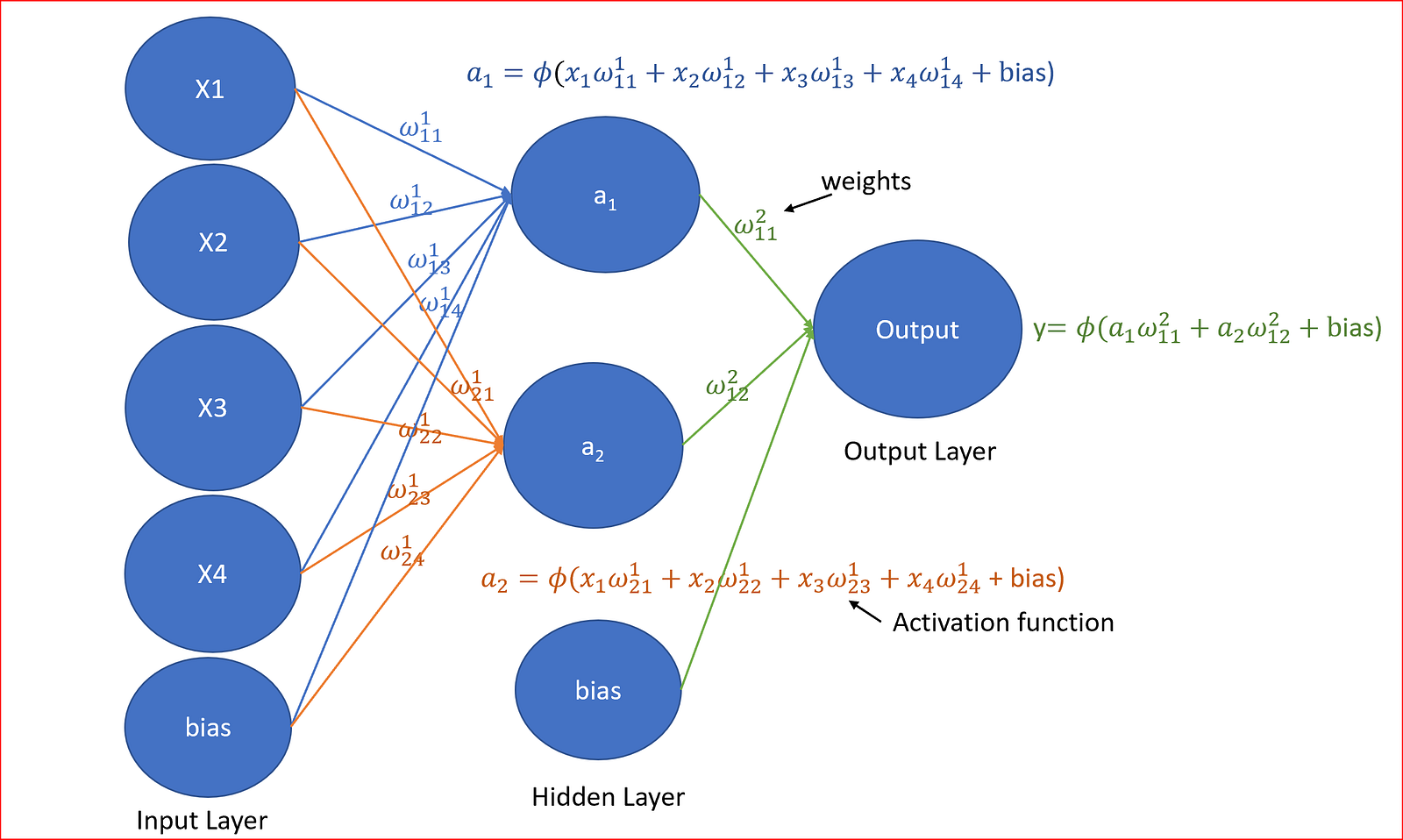Neural network with input, hidden and output layers along with activation unit

what are these weights for , what is activation function and what are these complex equations?

let’s simplify things

Weights are how neural networks learn. We adjust the weights to determine the strength of the signal.

weights helps us come up with different outputs.

For example, to predict a sunny day, temperature could anything between pleasant to hot, visibility is very good on a sunny days, so weights for temperature and visibility would be higher.

Humidity is not too high else it will be a wet day, so it could be that weight for humidity will be less or may be negative.

Wind speed may not have anything to do with sunny day it’s strength will be either 0 or very less.

we randomly initialize the weights(w) and multiply them with the inputs(x) and add the bias term(b), so for the hidden layer, a compact version is to calculate z and then apply the activation function(ɸ).

we call this Forward propagation. A compact generalized equation can be represented as shown below where l is no. of layer. For input layer l=1.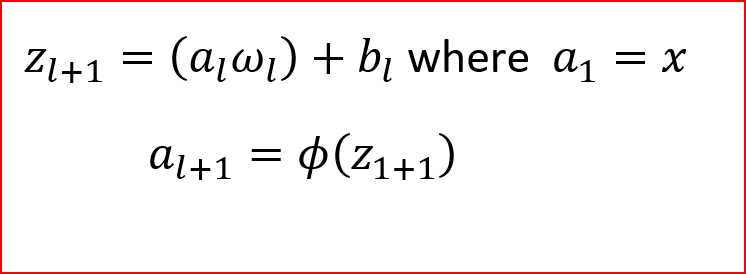Compact equation for forward propagation

Coming to activation functions, let’s understand what are they used for?

Activation function helps decide if we need to fire a neuron or not and if we need to fire a neuron then what will be the strength of the signal.

Activation function is the mechanism by which the neuron process and passes information through the neural network.

For a better understanding of different activation functions read my blog here

let’s understand the neural network with a sample data for predicting weather

To simplify things for a better understanding, we will just take two inputs : temperature and visibility with 2 hidden nodes, no bias units and we still want to classify the weather as sunny or not sunny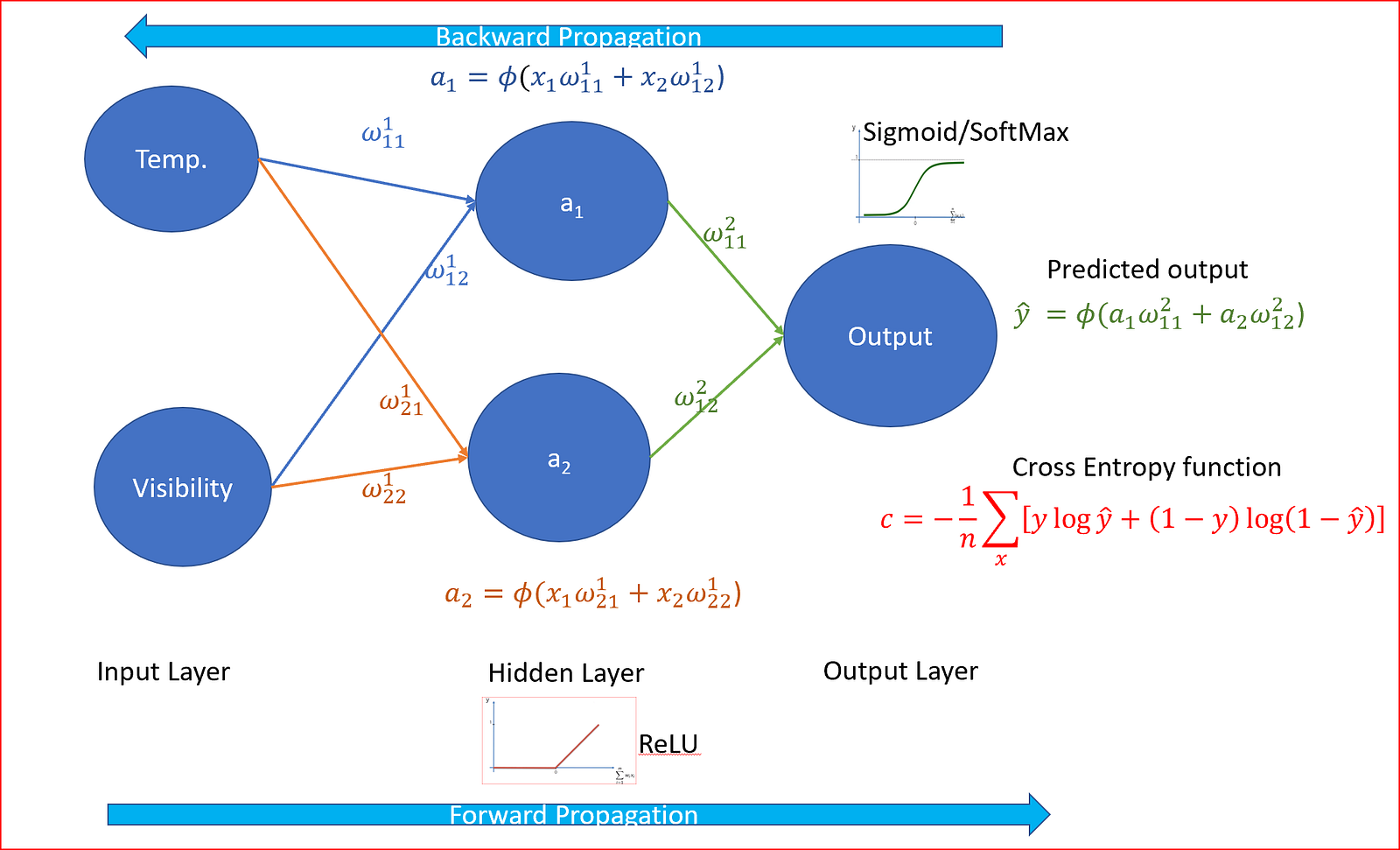Full neural network

we have temperature in fahrenheit and visibility is in miles.

let’s take a single data where temperature is 50F and visibility is .01 miles.

Step 1: we randomly initialize the weights to a value close to zero but not equal to zero.

Step 2: Next we take our single datapoint with our input nodes of temperature and visibility and move through the neural network.

Step 3: Apply forward propagation from left to right multiplying the weights to the input values and then using ReLU as the activation function. we know that ReLU is the best activation function for hidden layers.

Step 4: we now predict the output and compare predicted output with the actual output value. Since this is a classification problem we use cross entropy function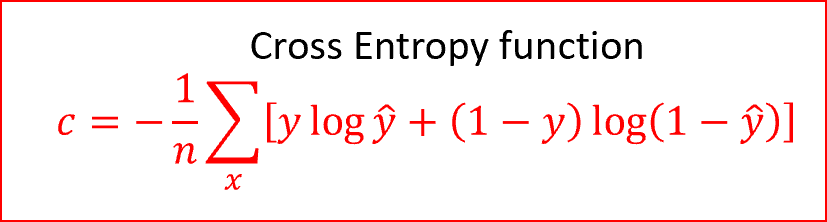n is total number of data points in the dataset, summing over all the inputs in the training set, y is the actual output and y ̂ (y-hat)is the predicted output

Cross Entropy is a non negative cost function and the range is between 0 and 1

In our example, the actual output is not a sunny day, so value of y will be 0. If y-hat is 1 then let’s substitute the values in the cost function and see what we get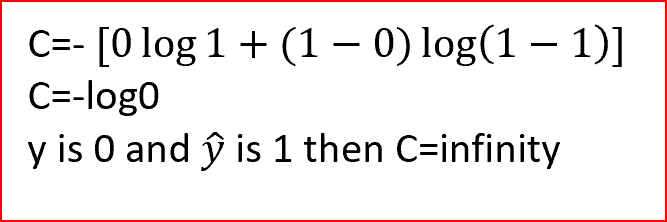predicted output is different than actual output

similarly when the actual output and the predicted output is same we get the cost c=0.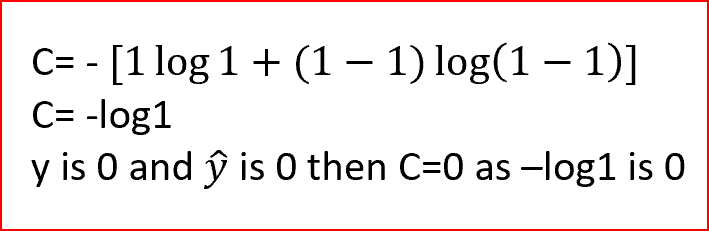predicted output is same as actual output

we can see for cross entropy function, when predicted output matches the actual output then cost is zero. When predicted output does not match with actual output then the cost is infinity.

Step 5: we do back propagation from right to left and adjust the weights. Weights are adjusted according to how much the weights are responsible for the error. Learning rate decides how much we update the weights.

so many jargons back propagation, learning rate. we will explain everything is simple terms.

#### Back propagation

Think of back propagation as a feedback mechanism that we sometimes get from our parents, mentors, peers. Feedback helps us become a better person.

Back propagation is a fast algorithm for learning. It tells us how the cost function will change when we change the weights and biases. Thus changing the behaviour of the neural network.

Without going into the detailed mathematics for backward propagation. In back propagation, we compute the partial derivative of the cost with respect to weight and partial derivative of the cost with respect to biases for each training example. Average the partial derivatives over all the training examples.

For our single data point, we determine the amount each of the weights and biases was responsible for the error .Based on how the weights are responsible for error, we adjust the all weights simultaneously.

Weights can be updated once for all of the training data using Batch gradient descent(GD) or once for each of the training example using Stochastic gradient descent(SGD).

we repeat step 1 through step 5 for different weights using either GD or SGD.

As the weights gets adjusted, certain nodes will be turned on or off based on the activation function.

in our weather example, temperature may have less relevance to predict a cloudy as the temperatures can be 70+ in summer and still cloudy or temperature can be 30F or less on a cold winter day and still be cloudy. In that case the activation function can decide to turn off the hidden node responsible for temperature and turn on only the visibility node to predict the output as not a sunny day as shown below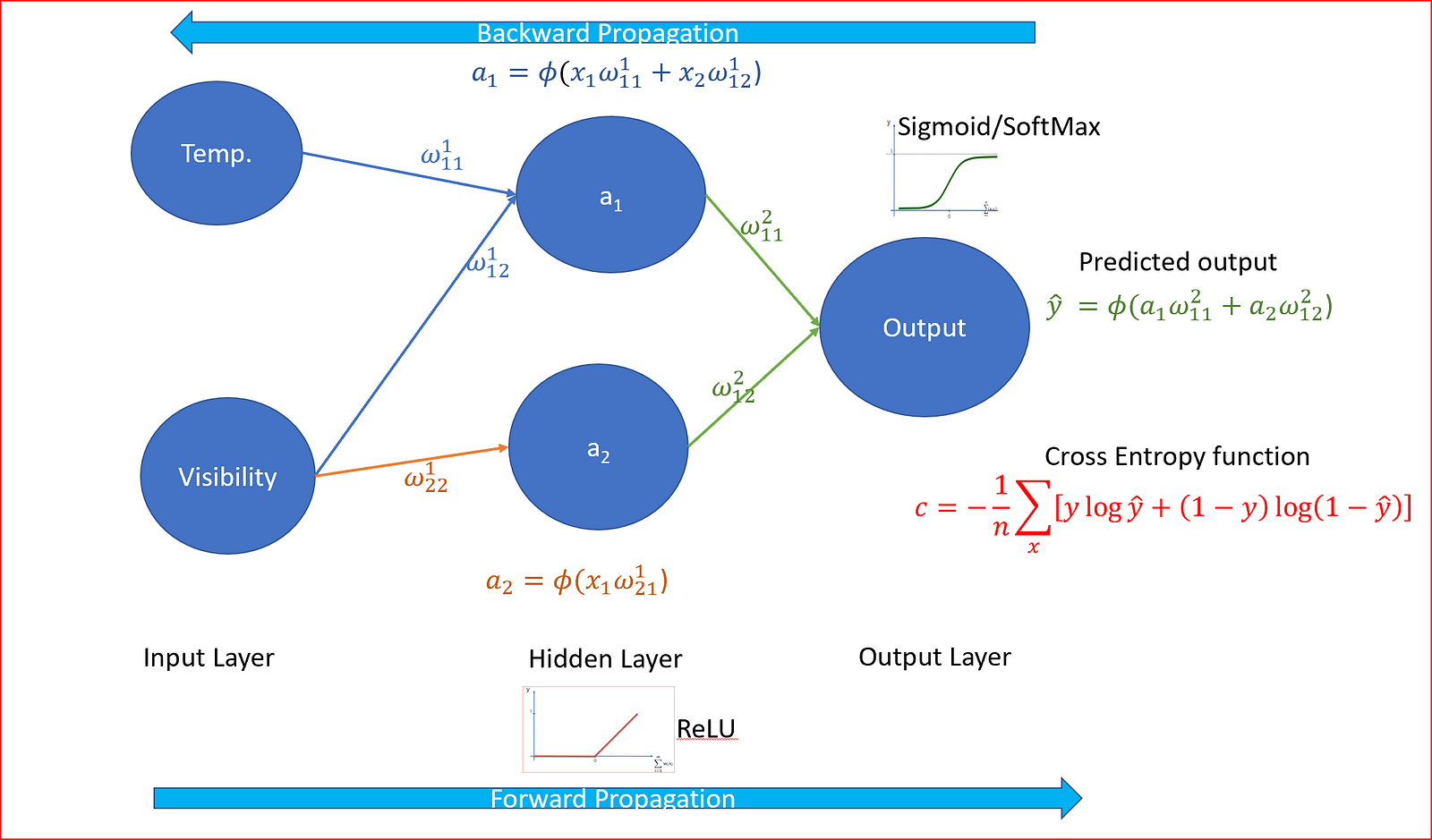weight for temperature is turned off for the second node for not sunny weather prediction

For more on Gradient Descent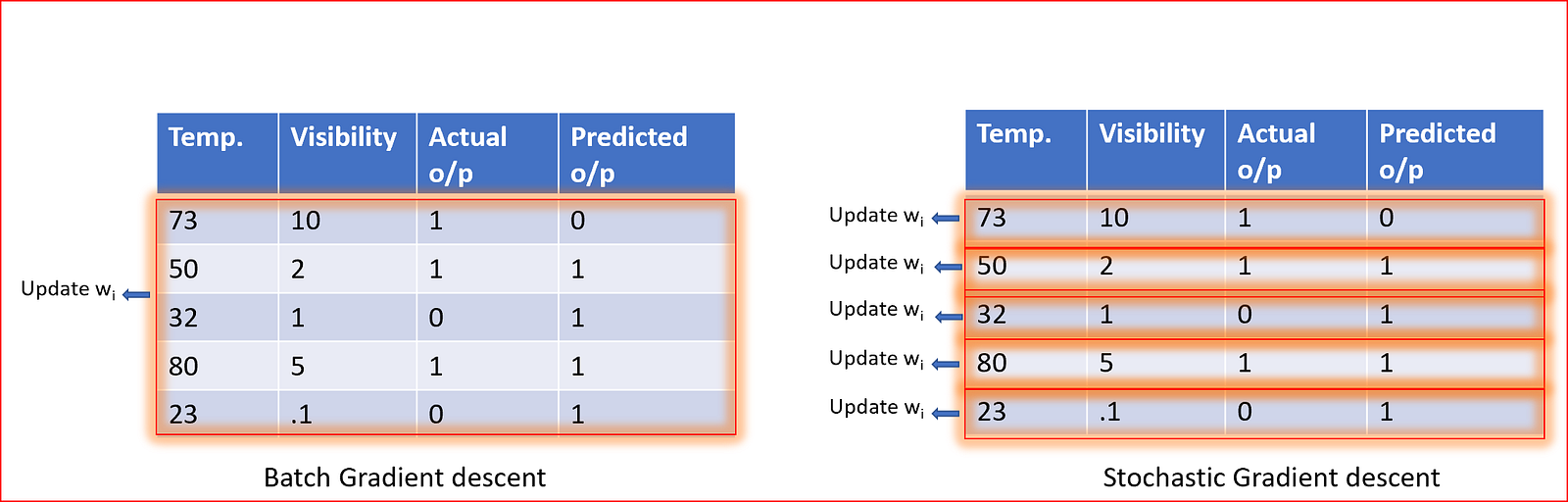Batch GD updates weight after each epoch. SGD updates weights for each training example

Epoch is when the complete dataset is used once for learning, one forward propagation and one backward propagation for all training example.

we repeat the forward and backward propagation for multiple epochs till we converge to a global minima.

#### What is learning rate?

Learning rate controls how much we should adjust the weights with respect to the loss gradient.

Lower the value of the learning rate, slower will be the convergence to global minima.

A higher value for learning rate will not allow the gradient descent to converge

Learning rates are randomly initialized.

How to decide on number of hidden layers and number of nodes in each hidden layer?

As we increase the number of hidden layers and number of neurons or nodes in hidden layer, we increase the capacity of the neural network. Neurons can collaborate to express different functions. This can often lead to overfitting and we must be careful of overfitting and underfitting.

For optimal number of hidden layers in a neural network is based on the following table as suggested by Jeff Heaton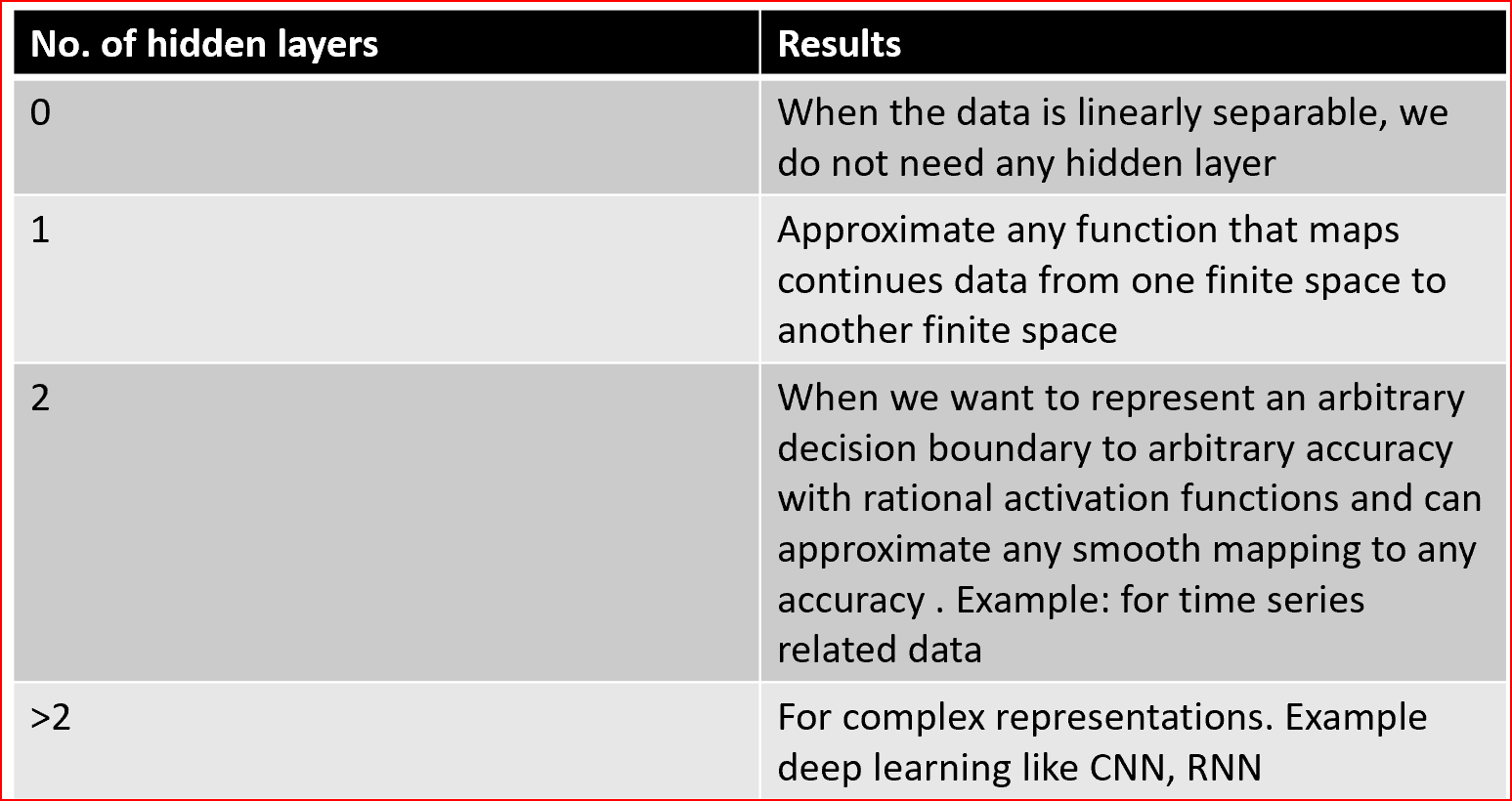Source: www.heatonresearch.com

For the optimal number of neurons in the hidden layer, we can follow any of the below approach

• Mean of the number of neurons in the input and output layer.
• Between the size of the input layer and the size of the output layer.
• 2/3 the size of the input layer, plus the size of the output layer.
• Less than twice the size of the input layer.

This was an effort to explain artificial neural network in a simplistic way without diving into complex maths.